# Transformer Delta to single phase

jaus tail

## Homework Statement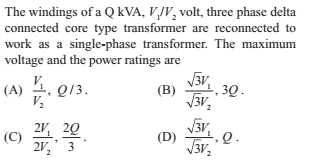## Homework Equations

For Delta line current = 1.732 times phase current.
Line voltage = phase voltage

## The Attempt at a Solution

I don't know how to start this.
This is original connection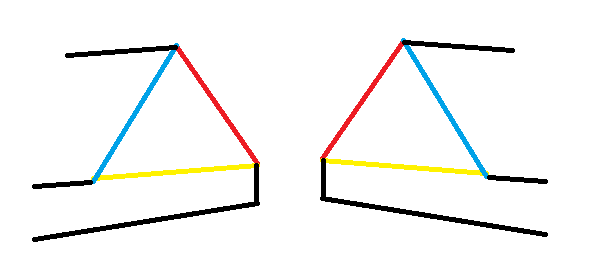This is new oneThe voltage should be 3 times because of additive polarity.

#### Attachments

How do you know that you must open the Δ to connect a single phase?

With the Δ connection, the three phasors sum to zero around the loop.
When you open the loop to connect the single phase and neutral, will you not be driving a short circuit?

•jaus tail
The core design matters, and based on what I can find, "Core type" is pretty ambiguous. Am I right on this?

•jaus tail
You will need context for the question. Maybe the text in the chapter the question came from will yield a clue.
A three phase transformer can be made from three single phase transformers each with two windings, or with less core material as a single three phase core with six windings.
Take a look at; http://www.electronics-tutorials.ws/transformer/three-phase-transformer.html

•jaus tail
jaus tail
There is no specific text before this question. There is a chapter on transformer followed by a questionnaire of 40 questions. In 3-phase there was: bank of 3 single phase transformer vs one three phase transformer. The clock nomenclature. Dy11, Yy0, etc... and parallel operation of transformers.

Since connecting three single phase transformers in parallel, for use as a 1PH transformer is trivial, you can assume the transformer has one 3PH core and so must be connected in Y, D or left open.

There are a couple of possibilities.
1. You might connect the A and N to two of the Delta terminals with one left open.
2. You might reconfigure the deltas to stars, then use centre neutral and one only of the three Y terminals for one phase.

Would either of those satisfy any of the possible answers?

•jaus tail
Also note - it is convention to use the diagram angles to represent the phase angles, so in your diagram, the color coding is helpful, but lay them out in a straight line . However this is only referring to the electical connections and phase angles.
In most 3 Phase transformers, if you do this ONE of the three windings needs to have opposite magnetic polarity than the other two, this affects the rating of the core, that is why the layout matters, and to me, maked the problem difficult without knowing the specifics of the core. -- If the problem is to be three separate transformers, then the problem is easier.

•jaus tail
jaus tail
I'm not understanding anything. Delta doesn't have a neutral. It's an equilateral triangle. Star has neutral for neutral current. That's why it's used for 1-phase load as well. Right? I tried this diagram.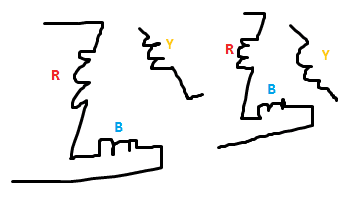V = Vr + Vy = Vr + Vr angle (120 degrees)
= Vr + Vr cos 120 + j Vr sin 120
= 0.5 Vr + j0.866 Vr
= Vr angle 60 degrees.

#### Attachments

If you drive a three phase transformer with only one single phase, there is no way you can generate more than that one phase and it's opposite polarity, at 180°.
So what would happen if you connected a neutral and active of a single phase to two of the three terminals of a delta primary? Would the equivalent delta secondary terminal pair produce a single phase output?

Consider redrawing your closed delta with one corner at the origin.

•jaus tail
jaus tail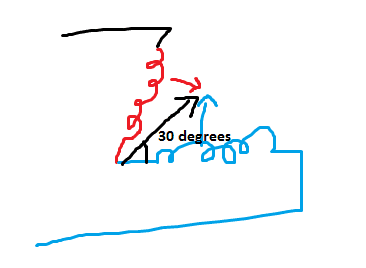Can it be this?
The voltage will be divided equally. So V = Vr/2 + Vr/2 angle 120 where Vr is phase voltage on R winding for 3-phase ckt.
= 0.25 + j0.433
= 0.5 angle 60
Drawing neutral. I have no idea what i just did. I know it's wrong.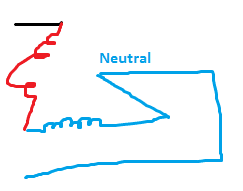Now what?

Well at least I got how the voltage rating becomes 2V. Since two windings are in series, they divide voltage equally. So the applied voltage can be 2V.
But how to go ahead for Power rating?

#### Attachments

You are still drawing the diagrams as having phase.

The three cores of the 3PH transformer share the magnetic circuits of the currents in the windings.
The field of each winding is split in two, with half passing through each of the other two windings.

jaus tail
Something like this?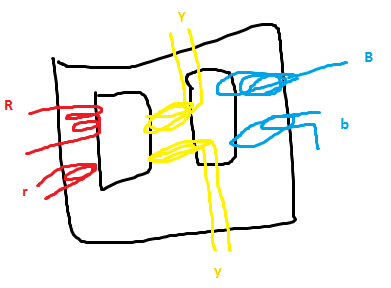Now I have 1-phase supply. Let terminals be L and N. So I connect L with R, other end of R with Y, other end of Y with N?
And same for LV side?